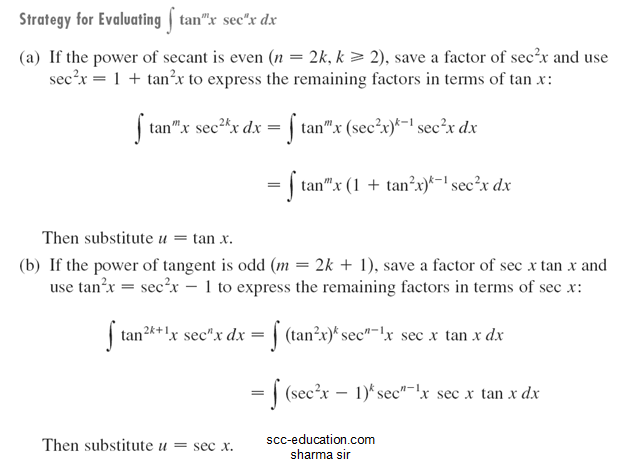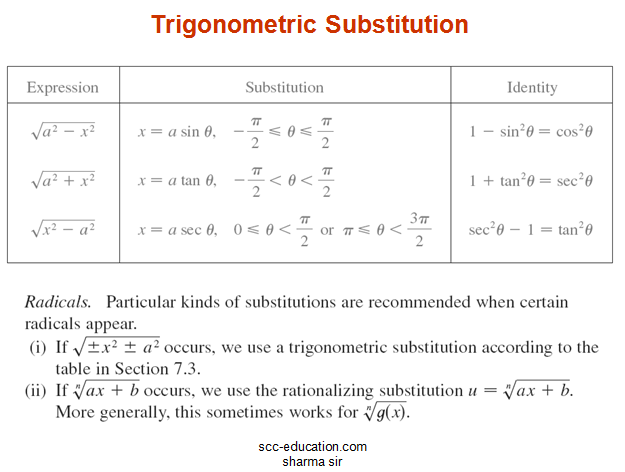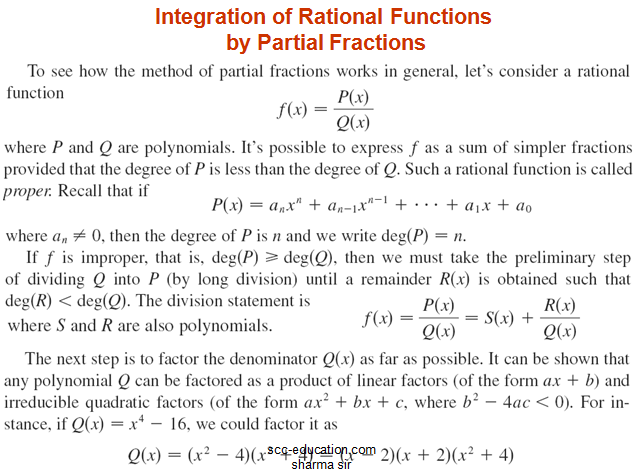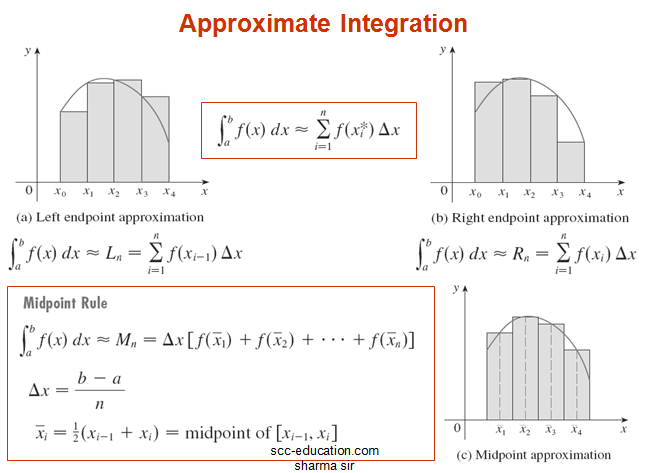# SCC Education

## Techniques of Integration •Substitution Rule •Integration by Parts •Trigonometric Integrals •Trigonometric Substitution •Integration of Rational Functions by Partial Fractions •Rationalizing Substitutions •The Continuous Functions Which Do not Have Elementary Anti-derivatives.

Techniques of Integration
Substitution Rule
Integration by Parts
Trigonometric Integrals
Trigonometric Substitution
Integration of Rational Functions by Partial Fractions
Rationalizing Substitutions
The Continuous Functions Which Do not Have Elementary Anti-derivatives.
Improper Integrals
Type I: Infinite Intervals
Type 2: Discontinuous Integrands
Approximate Integration
Midpoint Rule
Trapezoidal Rule

Simpson’s Rule
Strategy for Integration
1. Using Table of Integration Formulas
2. Simplify the Integrand if Possible
Sometimes the use of algebraic manipulation or trigonometric identities will simplify the integrand and make the method of integration obvious.
3. Look for an Obvious Substitution
Try to find some function               in the integrand whose                    differential also occurs, apart from a constant factor.
3. Classify the Integrand According to Its Form
Trigonometric functions, Rational functions, Radicals, Integration by parts.
4. Manipulate the integrand.
Algebraic manipulations (perhaps rationalizing the denominator or using trigonometric identities) may be useful in transforming the integral into an easier form.
5. Relate the problem to previous problems
When you have built up some experience in integration, you may be able to use a method on a given integral that is similar to a method you have already used on a previous integral. Or you may even be able to express the given integral in terms of a previous one.
6. Use several methods
Sometimes two or three methods are required to evaluate an integral. The evaluation could involve several successive substitutions of different types, or it might combine integration by parts with one or more substitutions.some-important-formulas-of-integration

solving-models-related-to-ordinary differential equation

differentiation-of-exponential-functions

area-between-curve

vector

application-of-derivatives

homogenous-differential-equation

inverse-trigonometry-questions

integration

integration-questions

Rolles-theorem-and-mean-value-theorem

Techniques-of-integration-substitution

critical-points-and-point-of-inflection

intermediate-value-theorem

### Sample paper Mathematics 12

............

#### 1 comment:

1.